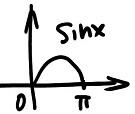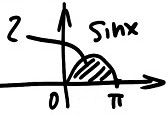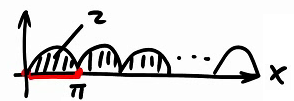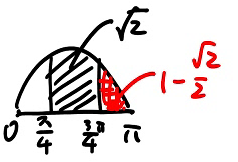### 一拱的定义### 一拱的面积

$int_{0}^{pi}sinxdx = -cosx|_0^{pi} = -(cospi - cos0) = -(-1-1) = -(-2) = 2$$cospi = -1$
$cos0 = 1$### 小结：★★★

1、$sinx$正弦曲线一拱的面积为$2$，那么半拱的面积就是$1$
2、记住下面常见部分的面积，作为常识使用：https://weibo.com/zhangyumaths